Scroll

# Average number of responses

Follow
 Summary This document provides detailed examples and explanations of how to use the average number of responses feature introduced in Analyse 5.3.3.5 Applies to Askia Analyse. Written for Data Processors and Analysts. Keywords Average, number, base, responses, calculation, multi-coded, normal, positive factor.

This calculation is used when the average number of responses given at a multi-coded variable is required. It can be used for both flat and cross calculations.

In the General Tab, right click > Insert in the calculations window: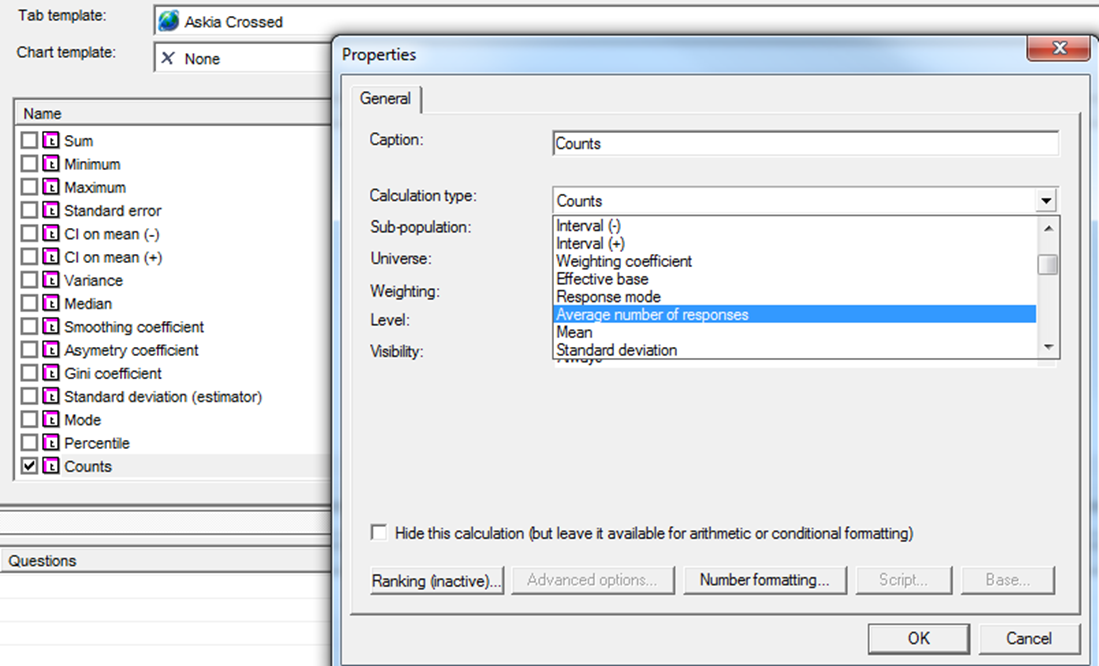Set the calculation type to be Average number of responses. There are some advanced options which will be explained below: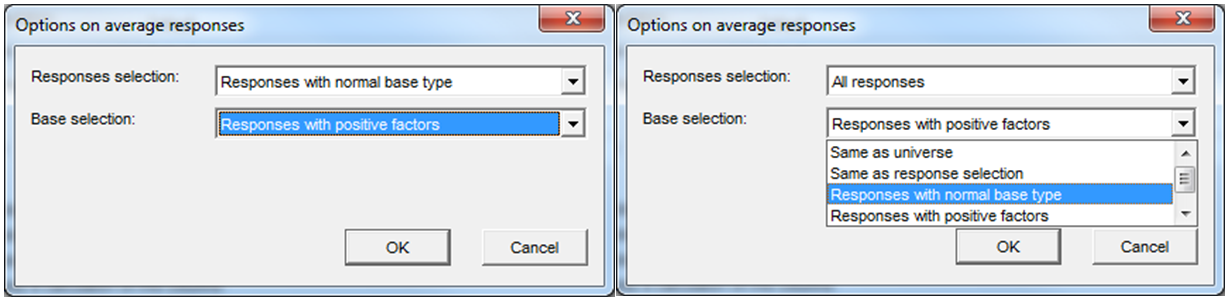Responses with normal base type are any responses that do not have their base type set to ‘Don’t know’ or ‘Not asked’.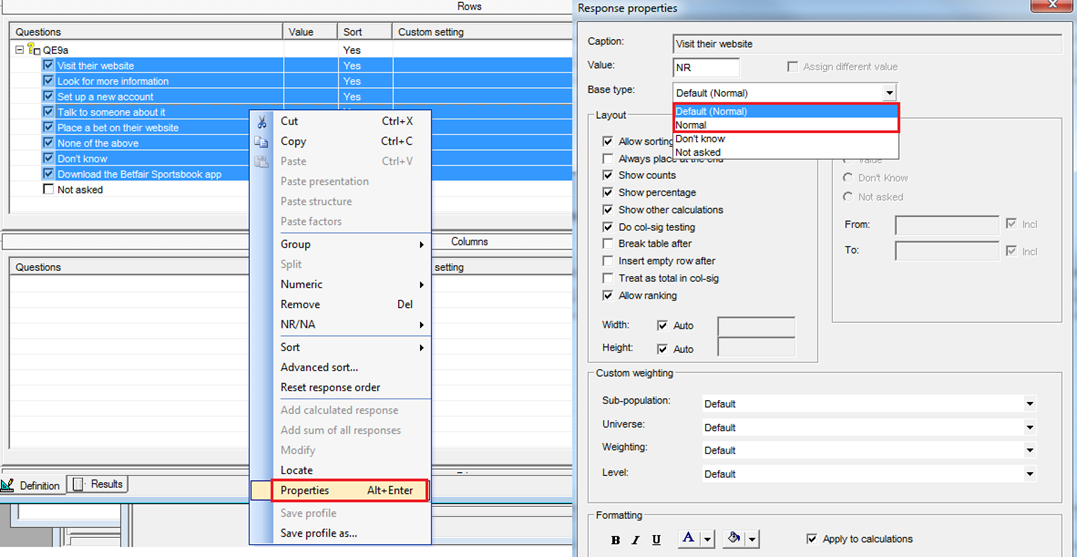Responses with positive factors:

In the variable properties select allow calculations for numerics.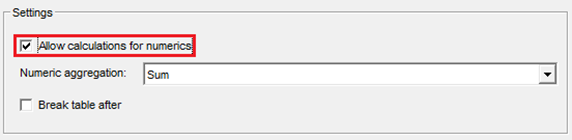1. You will see that your variable now has its Analysis values (factors) visible. They’re all set to NR as default (unless factors have been applied in Design).
2. Highlight the code whose factors you wish to update.
3. Copy any positive factors from Excel.
4. Right click on the codes you’ve highlighted > Paste factors (These can also be adjusted manually by right clicking on the responses > properties and amending Value to be 1 instead of NR)
5. You will see that factors are now applied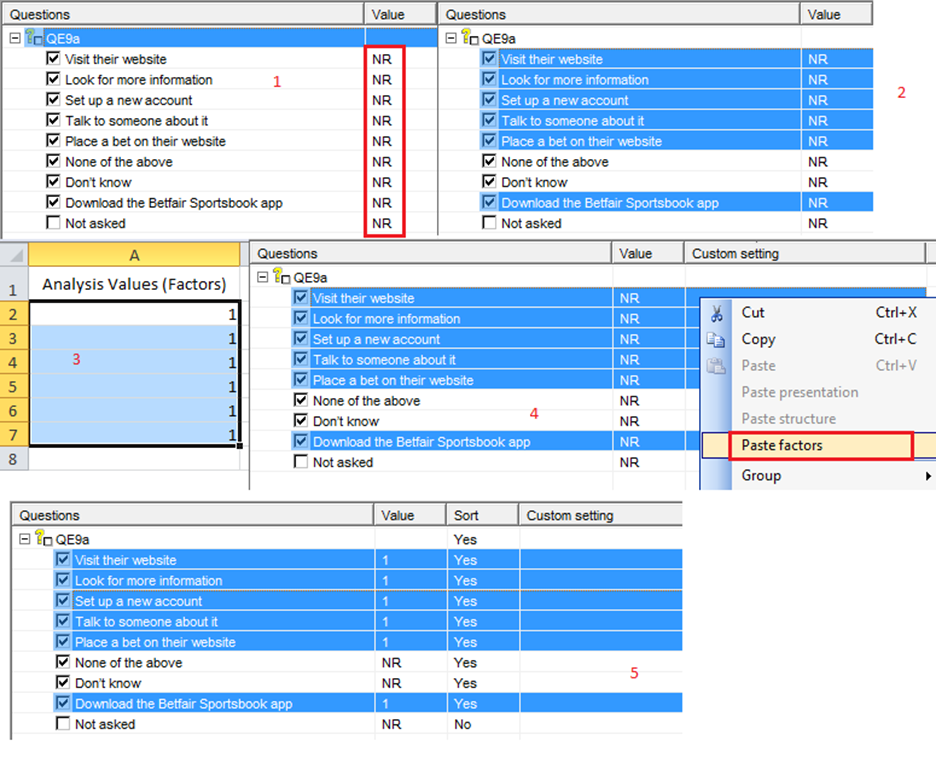So let’s take the example where we have the following advanced options:

A: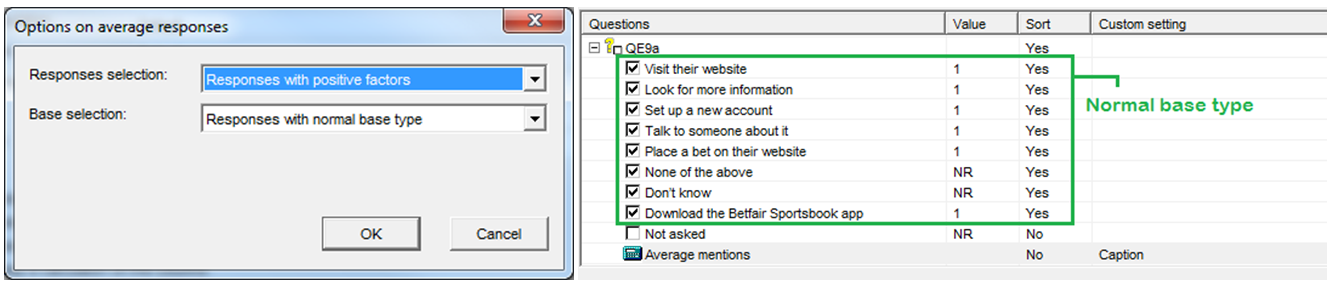Codes {1to5;8} have positive factors and codes {1to8} normal base type.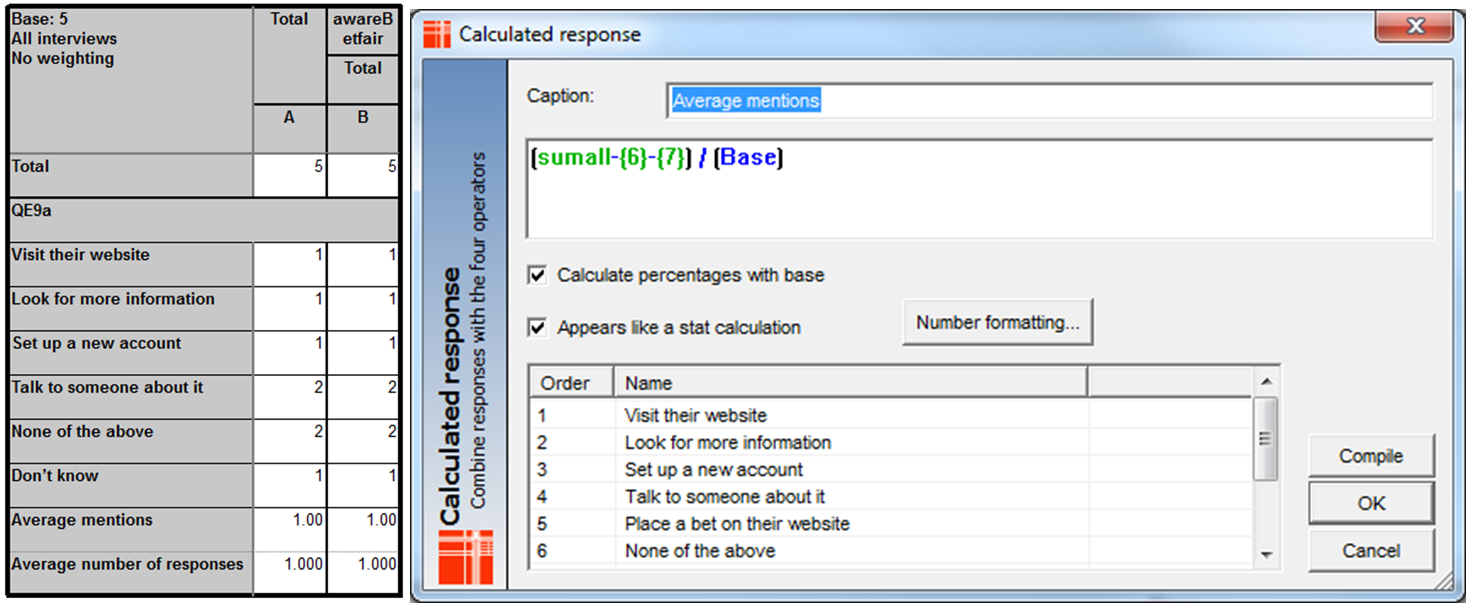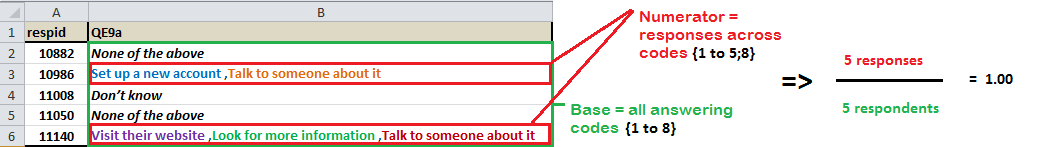So let’s another example where we have the same advanced options:

B: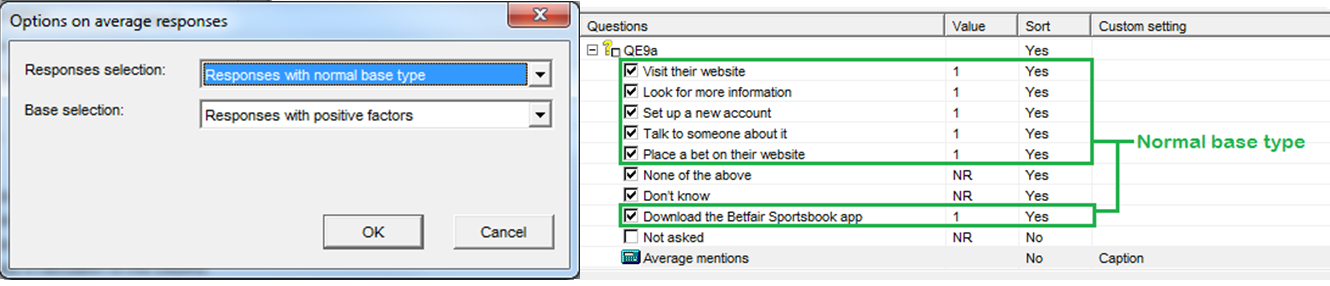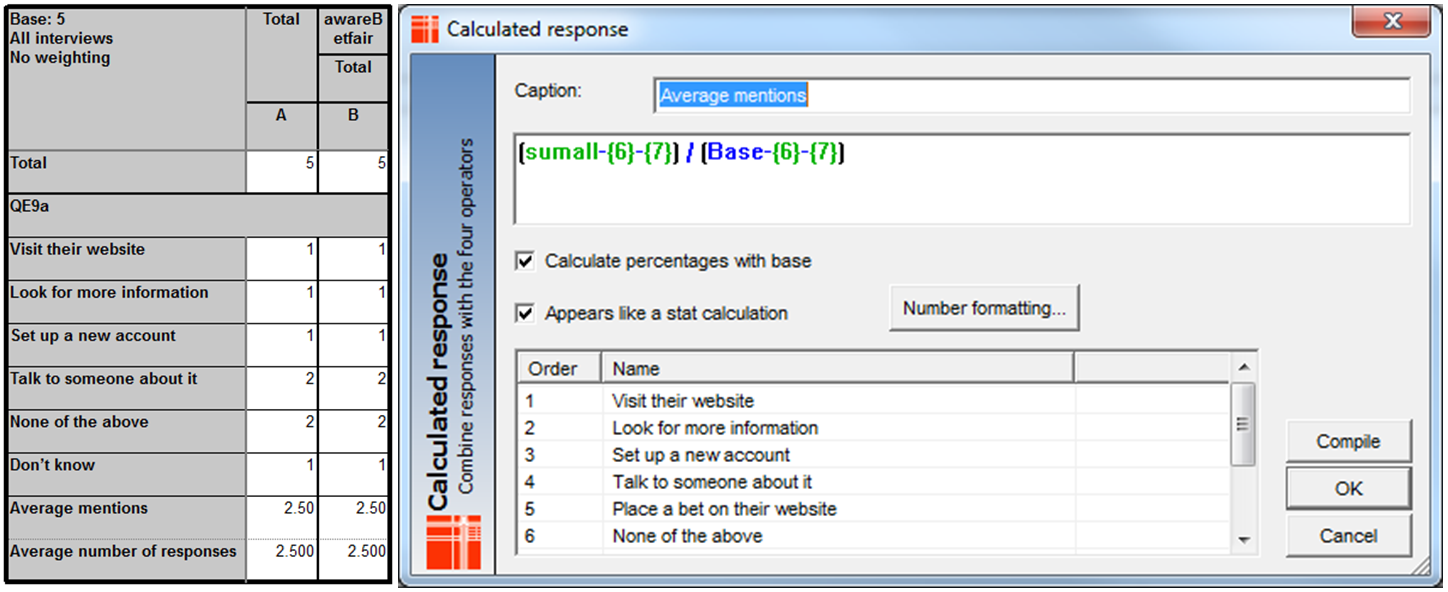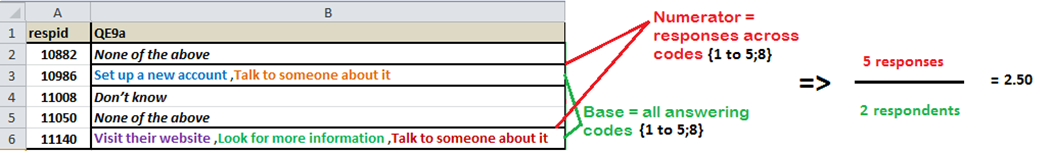These two examples should demonstrate, in a simple way, the advanced settings and how to interpret the results of this calculation. The test .qes file and portfolio can be downloaded from here.